Question 1:

Korea imports a large quantity of beef. With no beef trade, Korea’s equilibrium price for beef was \$8 million per kilo tonne and equilibrium quantity was 375 kilo tonne. If Korea opens its beef market to trade with no tariff, domestic demand would be 625 kilo tonne and domestic supply would be 125 kilo tonne at the world price of \$4 million per kilo tonne. However, Korea currently imposes 40 per cent tariff rate on all imported beef. With 40 per cent tariff, Korea’s domestic supply and domestic demand were 250 kilo tonne and 500 kilo tonne respectively in 2013. Assume that intercept of supply curve is \$2 million and demand curve is \$15 million per kilo tonne.

(a) Analyse the effects of 40 per cent tariff rate on the price, domestic supply and demand, and beef imports in comparison with no tariff case. Provide numeric details.

(b) Draw a graph and clearly show how the areas of gains and losses from the trade with 40 per cent tariff rate would change with brief explanation. Then, calculate the actual values of change in consumer surplus, producer surplus, tariff revenue and the amount of deadweight loss. Show your calculation.

(c) Suppose that Korea does not impose tariff any more but instead imposes an import quota of 250 kilo tonne. Draw a graph and show how the areas of gains and losses from the import quota would change. Provide your explanation.

Question 2:

(a) Terri runs a rose farm. The following table provides information on the number of workers and corresponding output. Complete the table for Terri’s marginal product and average product of labour schedules. Briefly explain how you calculate and show your calculation.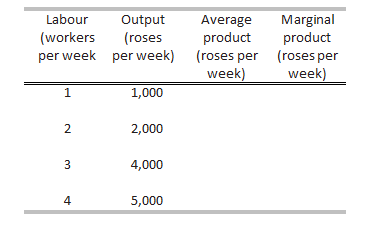(b) ProPainters hires students at \$250 a week to paint houses. It leases equipment at \$500 a week. The table sets out its total product schedule. Calculate and construct ProPainters’ cost schedules – that is, total cost (TC), average fixed cost (AFC), average variable cost (AVC), average total cost (ATC) and marginal cost (MC) per house painted. Briefly explain how you calculate each cost schedule and show your calculation.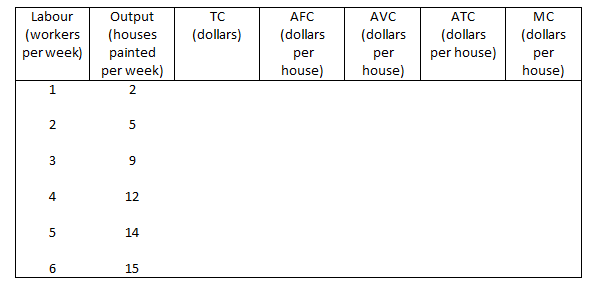Question 3:

The market for smoothies is perfectly competitive. The following table sets out the market demand schedule. Each of the 100 producers of smoothies has the costs given in the second table when it uses its least-cost plant.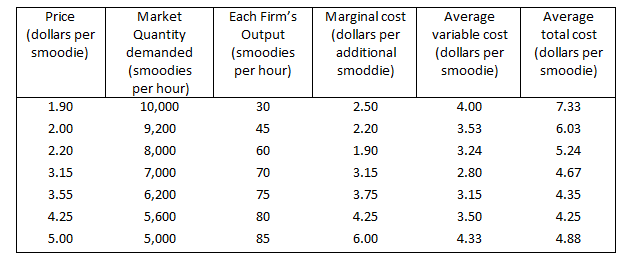(a) What is the market price and market output of smoodie in the short run and how many smoodies does each firm produce an hour? What is the economic profit made or economic loss incurred by each firm?  Explain and show how you calculated.

(b) What are the market equilibrium price and quantity demanded for smoodie in the long run? Do firms make economic profit or incur a loss? Explain why. Also, what will be the number of firms producing smoodie in the long run?

(a) The below figure highlights the above mentioned situation.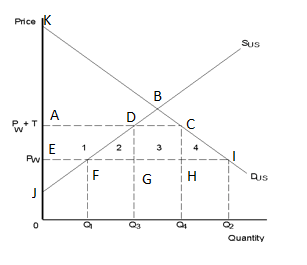As shown above the point B is the equilibrium quantity and price i.e. 375 kilo tonne and  \$8 million per kilo tonne.  If Korea opens its beef market to trade with no tariff, domestic demand would be 625 kilo tonne (Q2) and domestic supply would be 125 kilo tonne (Q1) at the world price of \$4 million per kilo tonne. The imports by Korea would be Q2- Q1 = 625 – 125 kilo tonne. Korea will be importing 500 kilo tonne beef from international market.

If Korea imposes 40 per cent tariff rate on all imported beef domestic supply (Q3) will be 250 kilo tonne and domestic demand (Q4) is 500 kilo tonne. The import of the nation will be Q4-Q3 = 500 – 250 kilo tonne. Thus Korea will be importing only 250 kilo tonne after imposing the tariff.

(b) The diagram shown above has been reproduced as shown below.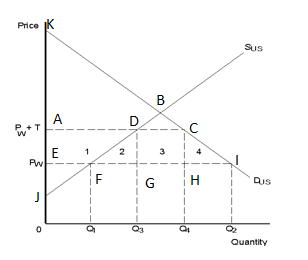The producer surplus in case no tariff is imposed is shown by the area bounded by JFE and after imposing the tariff the producer surplus will be area bounded by JDE. The difference in the two areas will be the producer surplus.

The area bounded by JFE will be

½ * (4-2)*(125-0) = 125

The area bounded by JDA will be

½ * (5.6-2)*(250-0) = 450

The producer surplus will be 275 (Shown by 1)

The consumer surplus in case no tariff is imposed is shown by the area bounded by KIE and after imposing the tariff the consumer surplus will be area bounded by KCA. The difference in the two areas will be the consumer surplus.

The area bounded by KIE will be

½ * (15-4)*(625-0) = 3437.5

The area bounded by KCA will be

½ * (15-5.6)*(500-0) = 2350

The consumer surplus will be -1087.5 (Shown by 1+2+3+4)

The tariff revenue for the government will be the area DCHG (Shown by 3). This will be

(500-250)*(5.6-4) = 400

Producer surplus + government revenue from tariff – Consumer surplus (Shown by 2+4)

275 + 400 – 1087.5 = -412.5

(c) In case Korea does not impose tariff any more but instead imposes an import quota of 250 kilo tonne, the graph will be similar to that shown above.In this case point A is the equilibrium price reached after the supply of 250million kilo tonne beef from international market. The producer surplus will be as shown by area ‘1’. Since there is no government revenue from tariff the deadweight loss will be area 2+3+4. Higher the equilibrium price higher will be the deadweight loss.

(a) Terri’s marginal product and average product of labour schedules has been shown below.

 Labour Output (roses Average Marginal product (workers per week) Product (roses per week) per week (roses per week) 1 1,000 1000 1000 2 2,000 1000 1000 3 4,000 1333.333333 2000 4 5,000 1250 1000

The average product is calculated based on the formula total product/ Input.

The marginal product is calculated based on the formula

(change in total product)/ (change in variable input)

It has been considered that is 0 labor is employed no output is there

(b) ProPainters’ cost schedules – that is, total cost (TC), average fixed cost (AFC), average variable cost (AVC), average total cost (ATC) and marginal cost (MC) per house painted has been shown below. Briefly explain how you calculate each cost schedule and show your calculation.

 Labour (workers Output (houses Variable Cost Fixed Cost TVC TC AFC AVC (dollars per house) ATC MC  (dollars per house) per week) Painted (\$ per worker) (\$ per week) (dollars) (dollars (dollars per week) per per house) house) 0 0 250 500 0 500 1 2 250 500 250 750 250 125 375 125 2 5 250 500 500 1000 100 100 200 83.33 3 9 250 500 750 1250 55.55 83.33 138.88 62.5 4 12 250 500 1000 1500 41.66 83.33 125 83.33 5 14 250 500 1250 1750 35.71 89.28 125 125 6 15 250 500 1500 2000 33.33 100 133.33 250

Total Cost (TC) is Total variable Cost + Total Fixed Cost

Average Fixed Cost (AFC) = Total Fixed Cost/ houses painted per week

Average variable Cost (AVC) = Total variable Cost/ houses painted per week

Average Total Cost (ATC) = Total Cost/ houses painted per week

Marginal Cost =(change in total Cost)/ (change in output).

(a) The average revenue is considered as the marginal revenue as in perfectly competitive market the price will remain constant. The equilibrium price is determined based on the demand and supply in the market. The equilibrium price is \$3.15 per smoodies and quantity is 7000 smoodies. The point at which the marginal cost cuts the marginal revenue curve is where the average total cost is \$4.67 per smoodie. Thus there will be economic loss which will be equal to the difference between the average cost and the marginal cost. This will be \$1.52per smoodie.

(b) In the long run the equilibrium point is where the marginal revenue equals marginal cost and the average cost. This is the point where the firms will not incur any profit or loss. As per the given information the equilibrium point is \$4.25 per smoodie. The quantity demanded is 5600 whereas there will be 80 smoodies produced by each firm. Thus there will be only 70 firms that will be producing total of 5600 smoodies.

OR

Explain Why Firmsâ€™ Marginal Cost Curves Become Upward Sloping After A Certain Level Of Output Has Been Reached.

Explain why firms’ marginal cost curves become upward sloping after a certain level of output has been reached. test While for the margina...

Tags: Australia Arlington Management Management University of New South Wales Management

Economics Assignment

Corrective Measures of Balance of Payment Deficits Balance of Payment (BoP) is a system followed by countries to keep an account of their income and expenditures. ...

Tags: Australia Arlington Management Management University of New South Wales Management

Economics Assignments

Introduction The assignment has two parts. The first part is related to the theories of micro economics. Micro economics analysis is based on the theory of law of ...

Tags: Australia Arlington Management Management University of New South Wales Management

Sports Economics

Introduction Every organization and every event needs to be structured properly for successful completion and evaluation. Whether it is business or sports, the app...

Tags: Australia Arlington Management Management University of New South Wales Management